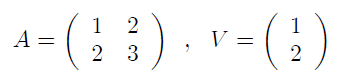# Solution Exercise 550 : numpy matrix product

#### Exercise 550

Given a square matrix A and a vector V:Give the Python code numpy which calculates the product AV (the matrix product and not the term to term product)

#### Solution

``import numpy as npA = np.array([[1,2],             [2,3]])V =  np.array([1,2])print(np.dot(A,V))``

Younes Derfoufi
my-courses.net